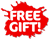Skip to content

# Casio fx-115ES PLUS Second Edition Advanced Scientific Calculator

by Casio
Condition: New
Sold out
SKU CASFX115ESPLS2S
\$36.99
\$36.99 - \$36.99
Current price \$36.99##### Item Description
• Use Cases: Algebra II, Trigonometry, Statistics, Calculus, Engineering, Physics
• Numeric Integration/differentiation: Simultaneous and polynomial solver
• Prime factorization: Matrix and vector calculations

## Overview

Casio's latest and advanced scientific calculator features Natural Textbook Display and advanced math functionality. The fx-115ES PLUS Second Edition has been designed to be the perfect choice for high school and college students

### Product Highlights:

Use Case: Algebra II, Trigonometry, Statistics, Calculus, Engineering, and Physics

Numeric Integration/differentiation: Simultaneous and polynomial solver

Prime factorization: Matrix and vector calculations

Complex number calculations: 40 Scientific constants

### Product Features:

LCD Display

Bright 16-digit display shows expressions and results in an easy-to-read format

Wide-Range of Uses

Over 280 functions, including fractions, statistics, complex number calculations, base arithmetic, linear regression, standard deviation, computer science, and polar-rectangular conversions

Multi-replay function allows you to backtrack step-by-step through calculations, where you can edit expressions and recalculate answers

Product Specifications:

GENERAL

Power Supply - Solar + (G13/LR44)x1

Display (Lines or Dots) - 16 x 4 Lines (96 x 31 Dots)

Auto Power OFF - Yes

DISPLAY / EXPRESSION

Natural Textbook Display - Yes

Input - Yes

Casio's latest and advanced scientific calculator features Natural Textbook Display and advanced math functionality. The fx-115ES PLUS Second Edition has been designed to be the perfect choice for high school and college students

### Product Highlights:

Use Case: Algebra II, Trigonometry, Statistics, Calculus, Engineering, and Physics

Numeric Integration/differentiation: Simultaneous and polynomial solver

Prime factorization: Matrix and vector calculations

Complex number calculations: 40 Scientific constants

### Product Features:

LCD Display

Bright 16-digit display shows expressions and results in an easy-to-read format

Wide-Range of Uses

Over 280 functions, including fractions, statistics, complex number calculations, base arithmetic, linear regression, standard deviation, computer science, and polar-rectangular conversions

Multi-replay function allows you to backtrack step-by-step through calculations, where you can edit expressions and recalculate answers

Product Specifications:

GENERAL

Power Supply - Solar + (G13/LR44)x1

Display (Lines or Dots) - 16 x 4 Lines (96 x 31 Dots)

Auto Power OFF - Yes

DISPLAY / EXPRESSION

Natural Textbook Display - Yes

Input - Yes

Output (Fraction) - Yes

Output (pi, root) - Yes

UNDO - No

TRANSFORMATIONS

Sexagesimal-Decimal Transformation - Yes

Engineering Notation - Yes

Recurring Decimal - Yes

BASIC FUNCTIONS

Trigonometric, Inverse Trigonometric - Yes

Hyperbolic, Inverse Hyperbolic - Yes

Exponental, Logarithmic (log,ln,10x,ex) - Yes

Power and Radical Root - Yes

Fraction - Yes

Mixed Fraction - Yes

Pi - Yes

Rounding - Yes

GCD/LCM - Yes

Remainder (R÷) - Yes

Base Specified Logarithmic (Log aB) - Yes

Prime Factorization - Yes

Ratio Calculation - No

UTILITIES

Variables 9

Variables List - No

Previous Answer Memory (Pre Ans) - Yes

Engineering Symbol Calculation - No

Solve function - Yes

CALC Function (Stored Formula Calculation) - Yes

Scientific Constant - 40

Metric Conversion - 40

SPECIAL FEATURES

Statistics - Yes

Standard Deviation - Yes

Regression - Yes

Variance - No

Stat Values List - No

Combination, Permutation (nCr, nPr) - Yes

Random Numbers - Yes

Random Integers (RanInt) - Yes

Integer / Nearest Lower Integer (Int,Intg) - Yes

Table Function - Yes

Type of Table - f(x), g(x)

Complex Number Calculation - Yes

Differentiation, Integration Calculation - Yes

Base-N Calculations - Yes

Matrix Calculations - Yes

Vector Calculations - Yes

Equation Calculation - Yes

Simultaneous Equation - Yes

Polynomial Equation - Yes

Storing Result - No

Inequality Calculation - Yes

Sum Calculation (SIGMA) - Yes

Product Calculation (Product of f(x)) - Yes

Verify Function - Yes

Distribution Calculation - No